# Trend Line In ExcelArticle byExcelMojo Team## What Is Trend Line In Excel?

The Trend Line in Excel is a line that provides a visual representation of the trend of the given dataset. It shows the existing data points’ trends, patterns, or directions. Since the Trend Line is not an inbuilt chart in Excel, we can plot it on some inbuilt charts that support it, such as Line Chart, Column Chart, Bar Chart, etc.

For example, the values of three items are in columns A & B.

We will create a Trend Line chart using the Column Chart from the “Insert” tab’s charts group. We get the Trend Line using the Column Chart, as shown below.

###### Key Takeaways
• The Trend Line in Excel is a dotted line plotted on an existing graph/chart to visualize the data points and the variation of data.
• We can plot a Trend Graph using different types of Trend Lines such as Linear, Exponential, Linear Forecast, etc., format it, extend the Trend Line into future or past periods, add multiple Trend Lines to the same chart, and remove Trend Line.
• A Trend Line is not a built-in chart in Excel but a built-in tool that is added from the Chart Elements on a generated chart.

### How To Add Trend Line In Excel?

Excel doesn’t have an inbuilt Trend Line chart. However, we can Create Trend Line in Excel using some of the chart types that support the same, such as Line Chart, Column Chart, Stock Chart, Bubble Chart, XY Scatter Chart, Area Chart, and Bar Chart.

We will Create Trend Line in Excel using a Line Chart’s “Line with markers” chart type with an example.

In the table, the data is,

• Column A shows the Item.
• Column B contains the Value.

The steps to create the Trend Line in Excel using the Line Chart’s “Line with markers” chart type are as follows:

1. Choose the data table → select the “Insert” tab → go to the “Charts” group → click the “Insert Line or Area Chart” option drop-down, as shown below.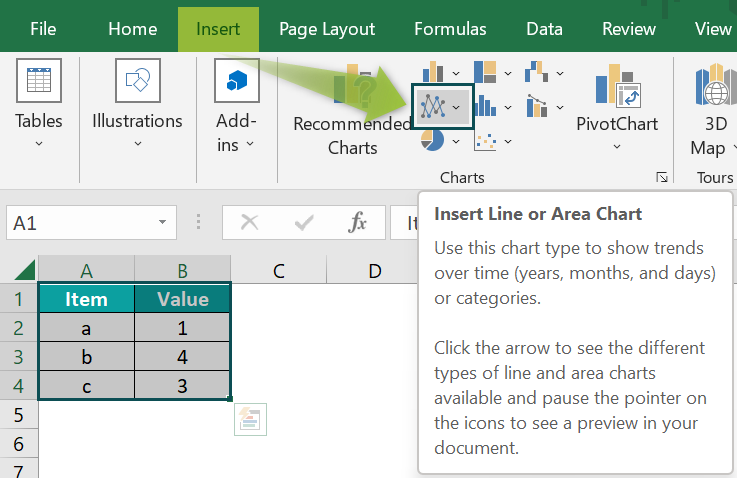2. Select the “Insert Line with Markers” chart type from the “2-D Line” category.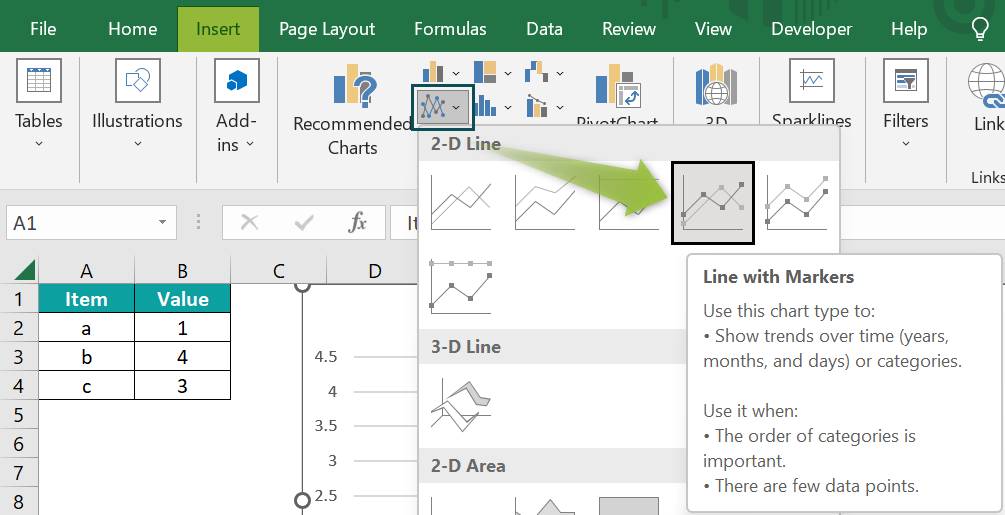The following chart is generated.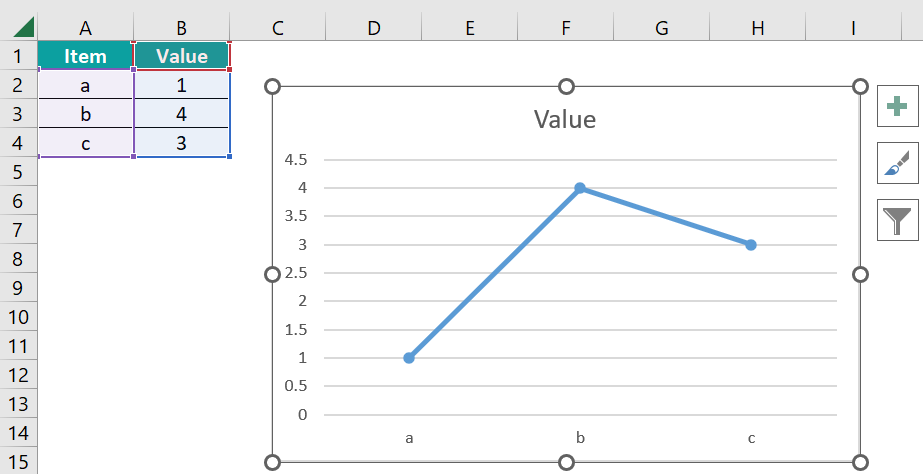3. Click anywhere on the chart to activate the formatting options.

Now, click the “+” symbol, i.e., the “Chart Elements” option → check/tick the “Trendline” options checkbox.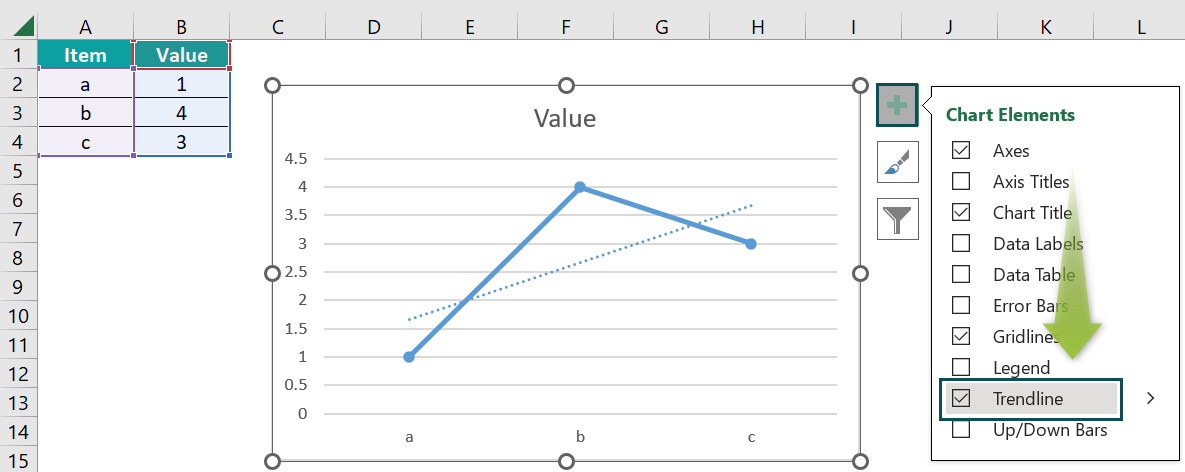The Trend Line, i.e., the dotted line through the graph, is formed for the given data values using the Line Chart’s “Line with markers” chart type, as shown above.

### Examples

We will create Trend Line in Excel using different inbuilt chart options.

#### Example #1

We will form the Trend Line in Excel using the Stacked Bar Chart in Excel for the given Quantity and Price of fruits.

In the table, the data is,

• Column A shows the Fruits.
• Column B contains the Quantity.
• Column C contains the Price.

The steps to create the Trend Line in Excel using the Stacked Bar Chart are as follows:

• Step 1: Choose the data table → select the “Insert” tab → go to the “Charts” group → click the “Insert Column or Bar Chart” option drop-down → select the “Clustered Bar” chart type, from the “2-D Bar” category, as shown below.

We get the following Bar Chart.

• Step 2: Click anywhere on the chart to activate the formatting options.

Now, click the “+” symbol, i.e., the “Chart Elements” option → click the “Trendline” options right arrow → select the “Linear” option, as shown below.

We get the Trend Line using the Stacked Bar Chart, as shown above.

#### Example #2

We will form the Trend Line in Excel using the Line Chart in Excel’s “Line” chart type.

For example, Column A contains the items, and columns B and C contains the sales and purchase of items, respectively.

The steps to create the Trend Line in Excel using the Line Chart’s “Line” chart type are as follows:

• Step 1: Choose the data table → select the “Insert” tab → go to the “Charts” group → click the “Insert Line or Area Chart” option drop-down → select the “Line” chart type from the “2-D Line” category, as shown below.

We get the Line Chart, as shown below.

• Step 2: Click anywhere on the chart to activate the formatting options.

Now, click the “+” symbol, i.e., the “Chart Elements” option → click the “Trendline” options right arrow → select the “Linear” option, as shown below.

We get the Trend Line using the Line Chart’s “Line” chart type., as shown above.

### Uses Of Trend Line In Excel

• The Trend Line is used when there is a certain rate of increase or decrease in data. For example, it is used to calculate the profit or loss of given data.
• When the Trend chart is plotted over any chart, it helps to visualize the points of the data variation.
• The Trend Line mathematically describes the line that best fits the data points when used in an equation or formula. The equations are different for different Trend Line types.

### Important Things To Note

• The Trend Line shows the “Moving Average” of the mentioned periods.
• We can always format the default Trend Line to make it more understandable.
• When we create a Trend Line for the chart, it automatically calculates the R-squared value.
• To know the most suitable Trend Line for your dataset, check for the R-squared value. When R-value is at or near 1, it’s most reliable.

1. What are the types of Trend Lines in Excel?

There are six types of Trend Lines as follows:
1) Exponential – The Trend Lines only work on the positive value of the data sets. It is used when the data increases or decreases at a quick rate.
2) Linear – The Trend Lines are best for simple data sets where the data points are in a line that increases or decreases at a steady rate.
3) Logarithmic – The rend Lines data increases or decreases; they are curved Trend Lines and can be used for charts with both positive and negative values.
4) Polynomial – The Trend Lines are curved lines for positive values of the given data.
5) Power – The Trend Lines are curved for data with positive values. Power Trend Lines are the best to use if your data increases at a specific rate.
6) Moving Average – The Trend Line is best if the data have large variations. They help neutralize variations to give you a better idea of the underlying trend.

2. How to Insert Trend Line in Excel?

The procedure to Insert Trend Line in Excel is,
First, select any chart from the Insert tab. Once the chart is generated, click on the chart to activate the “Chart Elements”.
Click the “+” symbol present to open the Chart Element list.
Check/Tick the Trend Line checkbox or select the type of Trend Line from the right arrow.
The Trend Line gets inserted, as shown in the below image.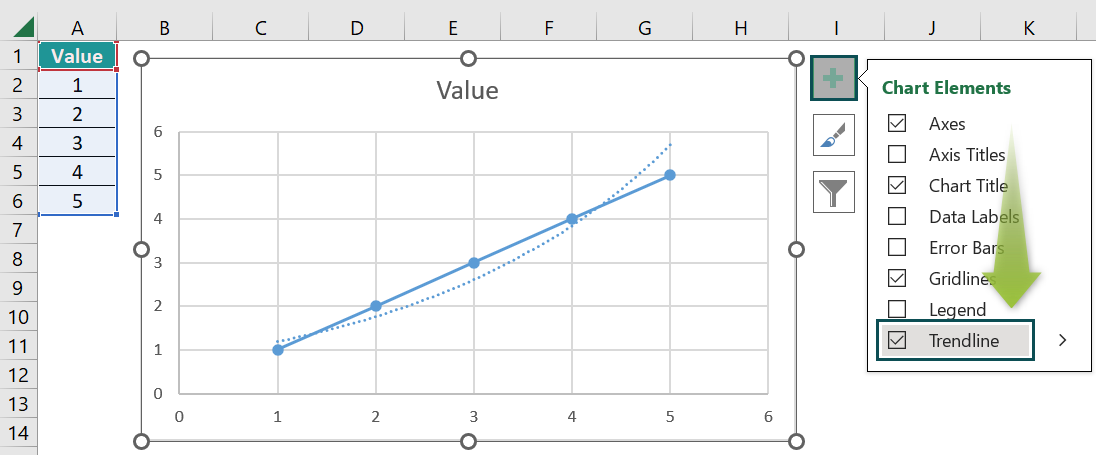3. Excel Chart Types for Trend Line?

The built-in Charts that support the Trend Lines in Excel are Line Chart, Column Chart, Stock Chart, Bubble Chart, XY Scatter Chart, Area Chart, and Bar Chart.

The built-in Charts that do not support the Trend Lines in Excel are Radar Chart, Pie Chart, 3D Chart, Stacked Chart, Surface Chart, and Doughnut Chart.